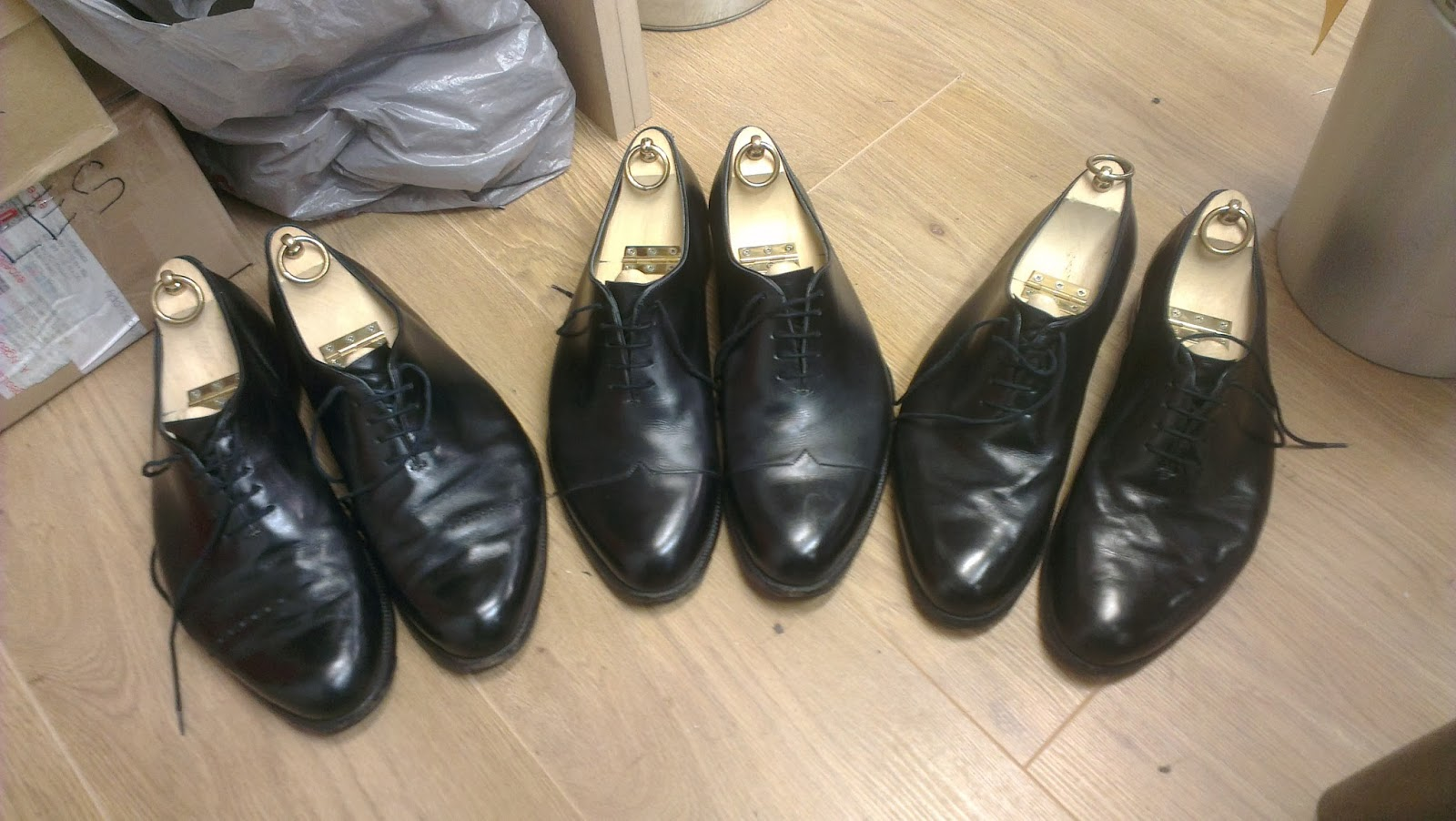# Would you mind if you wear unmatched shoes ?

Logic Level 2There are three pairs of shoes which are mixed up.It is impossible to separate the pairs visually or by any other way.However,the weights of any two shoes which belong to different pairs are different and weights of the shoes in the same pair are equal.

The weights of the pairs are light(L),medium(M) and heavy(H).Also,the sum of weights of a light shoe and a heavy shoe can be equal to sum of two medium shoes i.e.

$L+H=M+M$

If you are given an arm balance which is very accurate to even small weight differences then what is the minimum number of weighings you require to separate all the pairs?

Remarks:

Created in inspiration with this problem... Where is that hen?

×